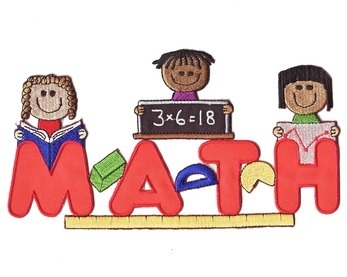# Math Vocabulary

•• ## STANDARDS FOR MATHEMATICAL PRACTICE THAT WE WORK ON CONTINUOUSLY THROUGHOUT THE YEAR ARE AS FOLLOWS:

1. Make sense of problems and persevere in solving them.
2. Reason abstractly and quantitatively.
3. Construct viable arguments and critique the reasoning of others.
4. Model with mathematics.
5. Use appropriate tools strategically.
6. Attend to precision.
7. Look for and make use of structure.
8. Look for- and express regularity in repeated reasoning.

• Chap. 5-Subtraction of Two-Digit Numbers
Vocabulary
Whole - Part = Part
Related Subtraction Facts
Subtraction Sentence
Count Up
Fact Family
Ones
Tens
Digit
2-Digit Number
Regroup
1 Ten = 10 Ones
Round
Estimate

Concepts

Subtract Tens and Ones
Mental Math Subtraction
Ways to Make Numbers
Regroup Tens as Ones: Use Models
Regroup Tens as Ones: Model and Record
Regroup Tens as Ones
Mental Math: Subtract Two-Digit Numbers
Estimate Differences
Rewrite Two-Digit Subtraction
Subtraction Practice
Chain Operations
Choose the Method
Mixed Practice
Problem Solving Applications: Mixed Strategies

Chapter 4

Vocabulary
Ones
Tens
Digit
Ten Rod
Two digit Number
Algorithm
Regroup
10 ones = 1 ten
Estimate
Round

Chapter 4 Concepts
Mental Math
Regroup Ones as Tens
Estimate Sums
Problem Solving Strategies

Chapter 3

Vocabulary
Pictograph
Bar Graph
Data
Symbol
Key
Survey
Range
Median
Mode
Predict
Line Plot

## Concepts Pictographs Bar Graphs Surveys Range, Mode, and Median Understanding Data Comparing Data Line Plots Problem Solving: Use a Graph Problem Solving Applications:Mixed Strategies

Chapter 2-Place Value to 100

Vocabulary
2-Digit Number
Place Value Chart
Expanded Form
Is Less Than (<)
Is Greater Than (>)
Is Equal To (=)
Estimate
Round
Halfway
Even Numbers
Odd Numbers
Count by 3s
Count by 4s
Ones Unit
Tens Rod
Hundred Flat
Value
Just Before
Just After
Between

Concepts
Tens and Ones
Place Value
Number Words 0-100
Place Value of 2-Digit Numbers
Expanded Form
Compare Numbers
Order Using a Number Line
Order Using Models
Estimate
Round to the Nearest Ten
Model Even and Odd
Even and Odd Numbers
Counting Patterns

• ## Chapter 1 - Adding & Subtracting to Twenty

• Chapter 1 Vocabulary
plus (+)
equals (=)
sum
make 10
doubles +1
doubles -1
subtract
minus (-)
difference
subtraction sentence
count up
fact family
part + part = whole
whole - part = part
doubles fact
related subtraction facts
fact family
•
• Chapter 1 Concepts

Extend Facts to 20
Doubles Facts
Doubles +1; Doubles -1
Subtraction Concepts
Count Back to Subtract
Related Subtraction Facts